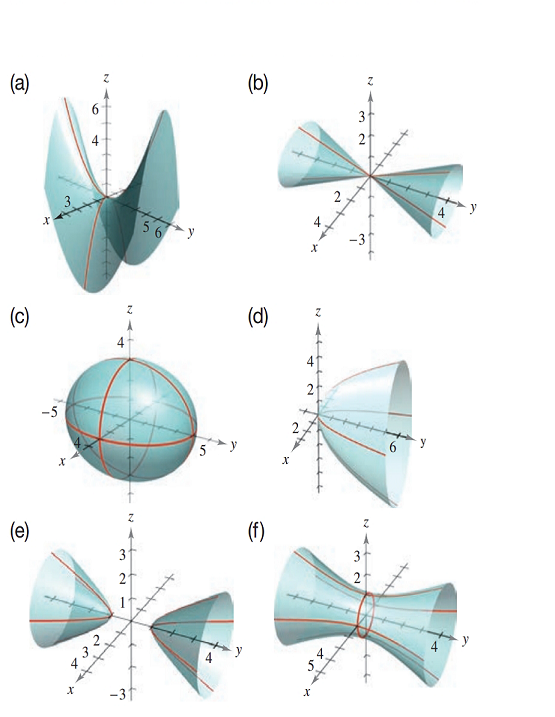Chapter 7.2, Problem 26E### Calculus: An Applied Approach (Min...

10th Edition
Ron Larson
ISBN: 9781305860919

#### Solutions

Chapter
Section### Calculus: An Applied Approach (Min...

10th Edition
Ron Larson
ISBN: 9781305860919
Textbook Problem

# Matching In Exercises 23-28, match the equation with its graph. [The graphs are labeled (a)-(f).]y 2 = 4 x 2 + 9 z 2

To determine

The quadratic surface represented by the equation y2=4x2+9z2 and its correct graph from the provided graph.

Explanation

Given Information:

The provided quadratic surface equation is y2=4x2+9z2 and the provided option for matching as follows:

Consider the provided equation y2=4x2+9z2

Rewrite the equation as in standard format,

y2=4x2+9z24x2+9z2y2=04x236+9z236y236=0x29+z24y236=0

The above equation can be written as,

x232+z

### Still sussing out bartleby?

Check out a sample textbook solution.

See a sample solution

#### The Solution to Your Study Problems

Bartleby provides explanations to thousands of textbook problems written by our experts, many with advanced degrees!

Get Started

#### Find more solutions based on key concepts